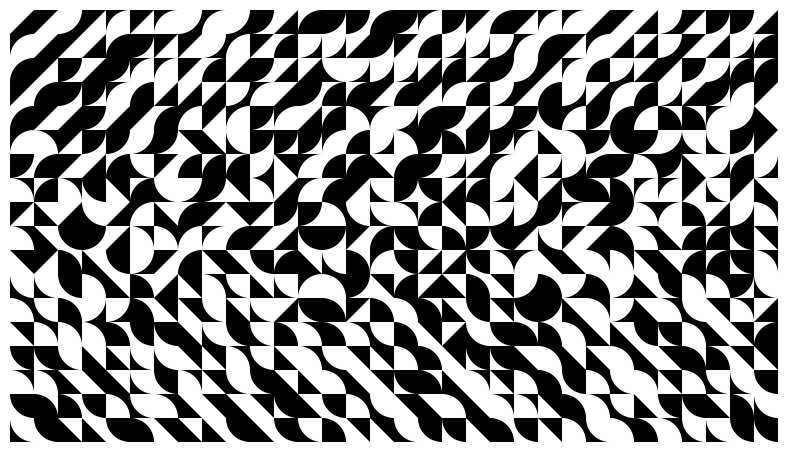# mathr / blog / #

## ReCode Project - Exploitation Quantitative...Had another look through the collection and implemented a variant on Exploitation Quantitative et Qualitative do Programme. I made the probabilites for the different cell shapes vary down the image, to get a vague leftwards pointing v shape.

import Diagrams.Prelude
import Diagrams.Backend.SVG.CmdLine (defaultMain)

import Data.Array (array, assocs, range)
import System.Random (StdGen, newStdGen, randoms)

main = newStdGen >>= defaultMain . diagram

diagram
= bg white . fc black . lwO 0
. flatten . grid . (randoms :: StdGen -> [Double])

flatten a = mconcat
[ c # centerXY # translate (r2 (fromIntegral i, fromIntegral j))
| ((i, j), c) <- assocs a
]

grid rs = array bs [ (ix, cell r ix) | (ix, r) <- range bs zip rs ]
where bs = ((0, 0), (sizeW, sizeH))

cell r = choose r . cells

choose r = snd . head . dropWhile ((< r) . fst) . cummulative

cummulative weighted =
let (weights, objects) = unzip weighted
weights' = scanl1 (+) weights
in  map (/ last weights') weights' zip objects

cells (i, j) =
[ (ascending , tri 0)
, (descending, tri 1)
, (ascending , tri 2)
, (descending, tri 3)
, (ascending , cir id 0)
, (descending, cir id 1)
, (ascending , cir id 2)
, (descending, cir id 3)
, (ascending , cir negate 0)
, (descending, cir negate 1)
, (ascending , cir negate 2)
, (descending, cir negate 3)
]
where
ascending  = (fromIntegral j / fromIntegral sizeH) ^ 2
descending = (fromIntegral (sizeH - j) / fromIntegral sizeH) ^ 2
corners k = take 3 . drop k . cycle $[ p2 (0, 0), p2 (1, 0), p2 (1, 1), p2 (0, 1) ] tri = strokeLoop . closeLine . fromVertices . corners cir f k = case corners k of [a,b,c] -> strokeLoop . closeLine$
arcBetween a c (f (1 - sqrt 0.5)) <> c ~~ b

sizeW, sizeH :: Int
sizeW = 32 - 1
sizeH = 18 - 1